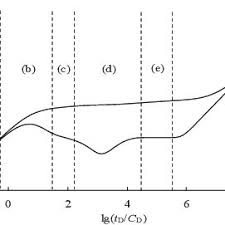## How to Calculate and Solve for Well Bore Storage Factor, Fluid Density and Well Annulus Cross-Sectional Area in Well Testing | The Calculator EncyclopediaThe image above represents the well bore storage factor.

To compute for the well bore storage factor, two essential parameters are needed and these parameters are Well Annulus Cross-Sectional Area (Ao) and Fluid Density (ρ).

The formula for calculating the well bore storage factor:

C = 144Ao / 5.615ρ

Where;

C = Well Bore Storage Factor
Ao = Well Annulus Cross-Sectional Area
ρ = Fluid Density

Let’s solve an example;
Find the well bore storage factor when the well annulus cross-sectional area is 54 and fluid density is 42.

This implies that;

Ao = Well Annulus Cross-Sectional Area = 54
ρ = Fluid Density = 42

C = 144Ao / 5.615ρ
C = 144 x 54 / 5.615 x 42
C = 7776/235.83
C = 32.97

Therefore, the well bore storage factor is 32.97.

Calculating the Well Annulus Cross-Sectional Area when the Well bore Storage Factor and the Fluid Density is Given.

Ao = C x 5.615ρ / 144

Where;

Ao = Well Annulus Cross-Sectional Area
C = Well Bore Storage Factor
ρ = Fluid Density

Let’s solve an example;
Find the well annulus cross-sectional area when the well bore storage factor is 34 and fluid density is 24.

This implies that;

C = Well Bore Storage Factor = 34
ρ = Fluid Density = 24

Ao = C x 5.615ρ / 144
Ao = 34 x 5.615 x 24 / 144
Ao = 4581.84 / 144
Ao = 31.81

Therefore, the well annulus cross-sectional area is 31.81.